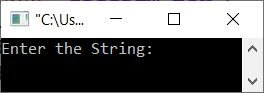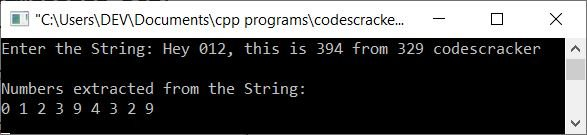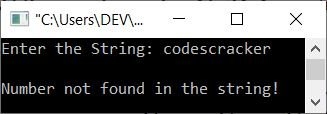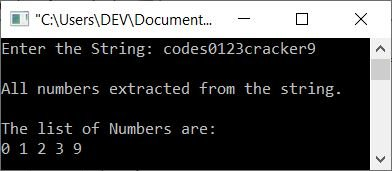# C++ Program to Extract Numbers from a String

This article provides some programs in C++ to extract all the numbers available in a string entered by the user at run-time. These are the lists of programs available in this article:

## Extract numbers from a string using a character array

The question is: write a program in C++ to extract and print numbers available in a string. The string must be entered by the user. The program given below is its answer:

```#include<iostream>
#include<stdio.h>
#include<string.h>

using namespace std;
int main()
{
char str, num;
int tot, i, j=0;
cout<<"Enter the String: ";
gets(str);
tot = strlen(str);
for(i=0; i<tot; i++)
{
if(str[i]>='0' && str[i]<='9')
{
num[j] = str[i];
j++;
}
}
cout<<endl<<"Numbers extracted from the String:"<<endl;
for(i=0; i<j; i++)
cout<<num[i]<<" ";
cout<<endl;
return 0;
}```

The snapshot given below shows the initial output produced by the above C++ program on extracting all the numbers available in a string entered by the user:Now supply the input, say Hey 012, this is 394 from 329 codescracker as a string. Press the `ENTER` key to extract and print all numbers available in the string as shown in the snapshot given below:## Extract numbers from a string using an integer array

This program does the same job as the previous program, but this program uses an integer array instead of a character array to do the job.

```#include<iostream>
#include<stdio.h>
#include<string.h>

using namespace std;
int main()
{
char str;
int tot, i, j=0, num;
cout<<"Enter the String: ";
gets(str);
tot = strlen(str);
for(i=0; i<tot; i++)
{
if(str[i]>='0' && str[i]<='9')
{
num[j] = str[i];
num[j] = num[j] - 48;
j++;
}
}
if(j==0)
else if(j==1)
{
cout<<"\nOnly one number found in the string:";
cout<<endl<<num;
}
else
{
cout<<"\nAll numbers extracted from the string.\n";
cout<<"\nThe list of Numbers are:"<<endl;
for(i=0; i<j; i++)
cout<<num[i]<<" ";
}
cout<<endl;
return 0;
}```

Here is its sample run with user input codescracker as a string:Here is another sample run with user input codes0123cracker9 as a string:Note that the statement num[j] = num[j] - 48 is used in the preceding program to convert the ASCII value to its equivalent character (a number).

Note: The ASCII value of 0 is 48, 1 is 49, 2 is 50, ..., and 9 is 57. This means that you can get the number by subtracting 48 from the ASCII value.

C++ Quiz

« Previous Program Next Program »